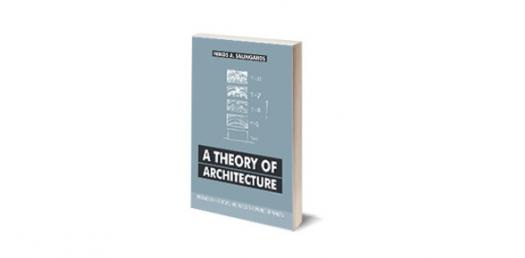# Quiz On A Theory Of Architecture Book

80 Questions | Attempts: 4149SettingsSubject: THEORY of ARCHITECTURE Answer the questions with the given choices. The entire questions are composed of basic and board exam type questions. Answer the questions sincerely with the time alloted. The main objective of the quiz is to let the examinees have the feel of answering questions while time pressured. Note: If you like to be notified for the updated questions and board exam events, add as on facebook: [email protected]/* */ If you have questions, feedback and suggestions regarding the quiz, email us at [email protected]/* */ Alternatively, you can share your feedback at archiTALK corner for your profesional insights and questions.

• 1.
A prismatic solid bounded by "six" equal square sides, the angle between any two adjacent faces being at right angle?
• A.

Cube

• B.

Unrecognizable form

• C.

Square

• D.

Hexagon

• 2.
Indicate a position in a space?
• A.

Line

• B.

Plane

• C.

Volume

• D.

Point

• 3.
A line extended in a direction other than its intrinsic direction?
• A.

Line

• B.

Plane

• C.

Volume

• D.

Point

• 4.
Defines the limit of a boundaries or volume in a creative composition?
• A.

Line

• B.

Plane

• C.

Volume

• D.

Point

• 5.
It is the primary identifying characteristic of a volume? Established by the shapes and interrelationships of the planes that describes the boundaries of the volume?
• A.

Texture

• B.

Form

• C.

Surface

• D.

Mass

• 6.
What architectural line is prominent in Prarie house designed by Frank Lloyd Wright?
• A.

Massiveness

• B.

Vertical

• C.

Horizontal

• D.

Combination of vertical and horizontal line

• 7.
The characteristic outline outline or surface configuration of a particular form and the principal aspect by which we identify form?
• A.

Texture

• B.

Form

• C.

Shape

• D.

Size

• 8.
The physical dimension of length, width and depth of a form. It's scale is relative to its other form in its context?
• A.

Proportion

• B.

Size

• C.

Shape

• D.

Mass

• 9.
A phenomenon of light and visual perception that may be described in terms of an individual's perception of hue, saturation and tone value. It distinguish a form from its environment?
• A.

Texture

• B.

Disaturation of surface

• C.

Color

• D.

Daylight

• 10.
An inclined line that rotates  360 degree having one end tip as a base of rotation?
• A.

Pyramid

• B.

Cone

• C.

Inclined cylinder

• D.

Plane

• 11.
A polyhedron having a polygonal base and triangular faces meeting at a common point or vertex?
• A.

Pyramid

• B.

Polygon

• C.

Cone

• D.

Hexagon

• 12.
All other forms can be understood to be a transformation of the primary solids, variations which are generated by the manipulation of one or more dimension or by the addition or subtraction of elements What type of form transformation in the figure shown above?
• A.

Dimensional transformation

• B.

Subtractive transformation

• C.

• D.

None

• 13.
What type of form transformation in the figure shown above?
• A.

Dimensional transformation

• B.

Subtractive transformation

• C.

• D.

None

• 14.
What type of form transformation in the figure shown above?
• A.

Dimensional transformation

• B.

Subtractive transformation

• C.

• D.

None

• 15.
The figure above was design by Adrea Palladio, identify which transformation of form it belongs?
• A.

Dimensional transformation

• B.

Subtractive transformation

• C.

• D.

None

• 16.
The figure above was design by Le Corbusier, identify which transformation of form it belongs?
• A.

Dimensional transformation

• B.

Subtractive transformation

• C.

• D.

None

• 17.
Identify which transformation of form is applied to the photo above?
• A.

Dimensional transformation

• B.

Subtractive transformation

• C.

• D.

None

• 18.
The figure above was design by Le Corbusier, identify which transformation of form it belongs?
• A.

Dimensional transformation

• B.

Subtractive transformation

• C.

• D.

None

• 19.
House at Stabio by Mario Botta, Identify which transformation of form is applied?
• A.

Dimensional transformation

• B.

Subtractive transformation

• C.

• D.

None

• 20.
By Le Corbusier comments on "forms", which of the composition that he mention is "very difficult" to satisfy the spirit?
• A.

Commutative composition

• B.

Cubic composition

• C.

Combination

• D.

Subtractive composition

• 21.
Under additive composition, what type of form  composition is "The 1 Mile High Illinois by FLW?
• A.

Linear form

• B.

• C.

Grid form

• D.

Clustered

• 22.
What type of form  composition is the secretariat building unesco headquarters paris?
• A.

Linear form

• B.

• C.

Grid form

• D.

Cluster

• 23.
What type of form  composition is the G.N. Black House?
• A.

Linear form

• B.

• C.

Grid form

• D.

Cluster of interlocking form

• 24.
Nakagin House by Kisho Kurokawa, identify what type of form  composition?
• A.

Linear form

• B.

• C.

Grid form

• D.

Cluster form

• 25.
Figure shown is the Habitat 67 by Moshe Safdie, identify what type of form composition?
• A.

Grid form

• B.

Linear form

• C.Back to top